Learning Library

Box-and-Whisker Plots

This collection of 10 worksheets that can help build a foundation for data analysis, graphing, and creating box-and-whisker plots.

Click on a worksheet in the set below to see more info or download the PDF.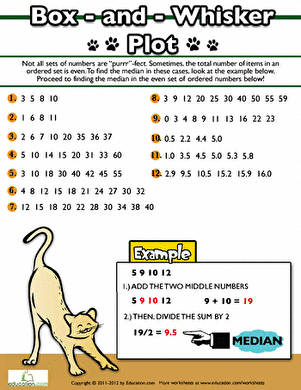How to Find the Median

Practice finding the median of a data set containing an even amount of numbers.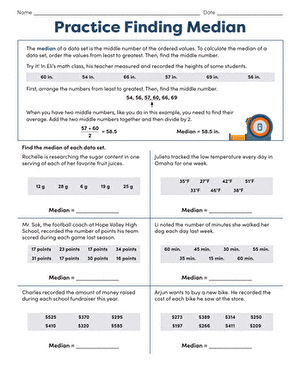Finding the Median

Introduce your math student to the basics of a box-and-whisker plot with this worksheet about finding the median.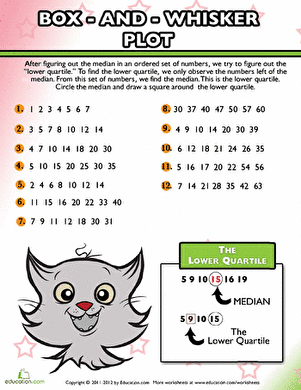How to Find the Lower Quartile

Learn the basics of creating a box-and-whisker plot with this worksheet about finding the lower quartile of a data set.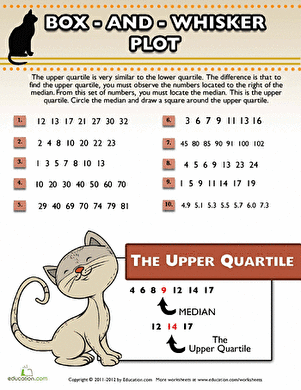How to Find the Upper Quartile

Build graphing and data analysis skills with this activity about finding the upper quartile. Students will see an example, then practice a few problems on their own.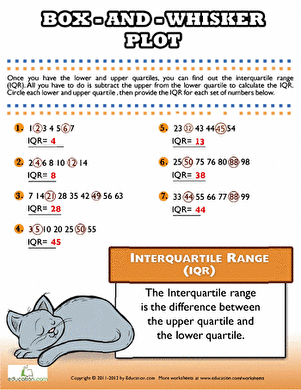Interquartile Range

There are many steps to graphing a box and whisker plot, including finding the interquartile range of the data set.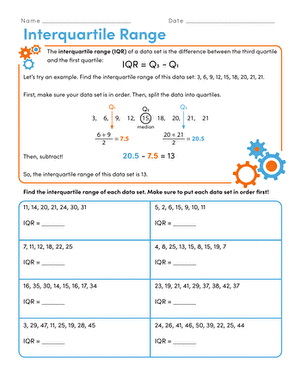Finding the Interquartile Range

For middle schoolers learning about box and whisker plots, here's a great worksheet all about finding the interquartile range of a data set.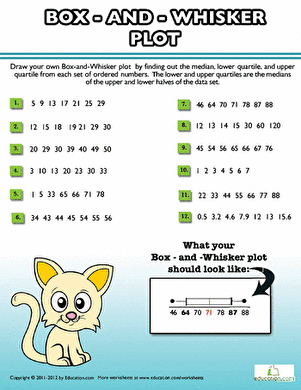Box and Whisker Plot Practice

Help your middle school student get a grasp on box and whisker plots with these practice problems that he can graph on a separate piece of paper.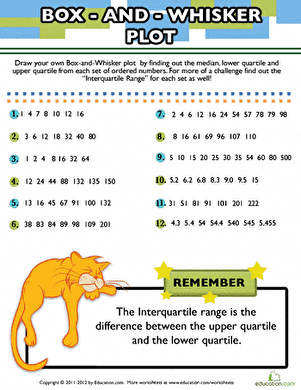Box and Whisker Plot: Data

Challenge students to analyze these data sets by using box-and-whiskers plots! Students will practice calculating the median and interquartile range.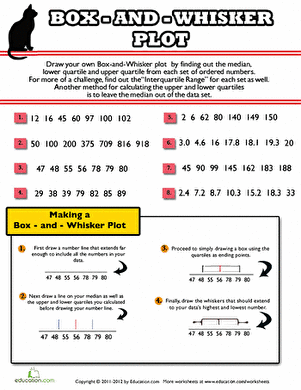Box and Whisker Plot Problems

Box-and-whisker plots are a breeze in this great beginner's worksheet. Your pre-algebra student can practice finding the median and quartiles of a data set.

Want to download this whole set as a single PDF?

Create new collection

0

New Collection>

0 items

What could we do to improve Education.com?

Please note: Use the Contact Us link at the bottom of our website for account-specific questions or issues.

What would make you love Education.com?